# purgeR tutorial

#### 2022-07-11

purgeR is a package for the estimation of inbreeding-purging genetic parameters in pedigreed populations. These parameters include the inbreeding coefficient ($$F$$), partial ($$F_{i(j)}$$), ancestral ($$F_{a}$$) and purged ($$g$$) inbreeding coefficients, as well as the total and expressed opportunity of purging ($$O$$ and $$O_{e}$$, respectively). Only genealogical records are required to estimate them, and all individual estimates will be stored in a dataframe. Thus, purgeR provides the raw material for subsequent analysis on inbreeding depression and genetic purging (see the ‘ip’ vignette for more detailed examples on this).

In addition, functions are also included for the pre-processing of pedigrees, and for the analysis of population diversity (e.g. effective population size), and the inference of time (e.g. number of equivalent to complete generations), bottlenecks (e.g. effective number of founders and ancestors, and founder genome equivalents) and fitness (e.g. breeding success and reproductive value), among others. All these functions are helpful to contextualize the demographic circumstances under which inbreeding and purging occur, as well as their consequences.

The next sections give a practical introduction to all functions contained in the purgeR package. The tidyverse R dialect is used throughout the tutorial, including the pipe operator %>%. Users unfamiliar with it are encouraged to read the introductory book R for Data Science.

## Basic input format

Most functions contain a mandatory argument ‘ped’, that will be used to input a dataframe with pedigree information. Pedigree dataframes need to follow some rules:

• Three columns are mandatory: individuals, mothers and fathers identities.
• Individuals must be sorted from older to younger.

To facilitate the usage of pedigrees and improve reproducibility, most functions will in addition require that columns for individuals, mother and fathers’ identities are named ‘id’, ‘dam’, and ‘sire’ respectively, all of type integer, with unknown parents named ‘0’. Individuals should in addition be named in order, from 1 to N.

There is no restriction to the addition of more columns, e.g. containing measures of individual genetic or environmental factors.

### Sort and rename individuals

Example pedigrees in this package are given already sorted, which means that ancestors are always placed on top of descendants. This is a requirement for all functions in the package, except for ped_sort, which is a function dedicated to sort individuals following Zhang et al. (2009) algorithm. See ?ped_sort for an example of use.

The function ped_rename is the most important pre-processing function in the package, and it will make sure that all input requirements are met, while making the changes needed for the remaining functions to work properly. Consider the example below using the pedigree of the Darwin/Wedgwood family:

data(darwin)
pander::pandoc.table(head(darwin))
Individual Mother Father
William Darwin I Unknown unknown
Mary Healey unk UNK
Gilbert Wedgwood 0 NA
Margaret Burslem 0 0
Anne Earle 0 0
William Darwin II Mary Healey William Darwin I

After using ped_rename, the pedigree is checked, and individuals are renamed in a proper format:

darwin <- purgeR::ped_rename(
ped = darwin,
id = "Individual",
dam = "Mother",
sire = "Father",
keep_names = TRUE
)
pander::pandoc.table(head(darwin))
id dam sire names
1 0 0 William Darwin I
2 0 0 Mary Healey
3 0 0 Gilbert Wedgwood
4 0 0 Margaret Burslem
5 0 0 Anne Earle
6 2 1 William Darwin II

Note that in the example only the first 6 rows are shown. In the renamed dataframe, Charles R. Darwin will appear with id = 52. Note as well the use of the option keep_names = TRUE. This will store the original individual identities on a separate column ‘names’.

### Reduce pedigree size

Downstream analyses may require at least one additional variable (column) containing some measurement of biological fitness (or any other value), meaning that individuals with no data available (i.e. with NA value) can be filtered out, as long as they are not ancestors of any other individual with available data. This is the job of the function ped_clean, that will reduce the size of the pedigree, and may improve the performance of inbreeding/purging functions in large pedigrees.

Taking as example the Dama gazelle pedigree (1316 individuals), ped_clean will reduce the pedigree size to 1176 individuals for the analyses of 15-days survival, and to 389 only when analyzing female productivity.

data(dama)
dama %>% nrow()
#>  1316
dama %>%
purgeR::ped_clean(value_from = "survival15") %>%
nrow()
#>  1025
dama %>%
purgeR::ped_clean(value_from = "prod") %>%
nrow()
#>  375

Note that ped_clean will require a renamed input pedigree. After its filtering step, it will automatically rename again the output pedigree.

## Inbreeding and Purging

Several measures of inbreeding and purging can be computed, based on the probability of allele identity by descent of individuals of the pedigree. All functions related to inbreeding and purging are prefixed with ip_.

### Wright’s inbreeding coefficient

The inbreeding coefficient ($$F$$, Wright 1922), here also referred to as standard inbreeding, is defined as the probability that an individual inherits two alleles derived from the same ancestor (i.e. identical by descent, IBD). In pedigreed populations, this can be calculated for an individual $$i$$ as the kinship coefficient of its parents $$j$$ and $$k$$ ($$F_{i} = f_{j,k}$$), which can be calculated as:

$f_{j,k {} (j=k)}=\frac{1}{2}(1+F_{j})$

$f_{j,k { } (j\neq k)}=\frac{1}{2}(f_{j,k_{d}}+f_{j,k_{s}})$ Where $$k_{d}$$ and $$k_{s}$$ refer to $$k$$’s dam and sire (see Falconer & Mackay 1996).

The function ip_F computes the inbreeding coefficient, given an input pedigree. Note that the value of $$F$$ will be saved in a new column of the dataframe, as it is usually convenient to save it this way to simplify the computation of further inbreeding and purging parameters, as well as for later analyses.

The example below shows the inbreeding coefficient of William E. Darwin (son of Charles R. Darwin and Emma Wedgwood).

darwin <- darwin %>% purgeR::ip_F()
darwin %>% dplyr::filter(names == "William Erasmus Darwin")
#>   id dam sire                  names         Fi
#> 1 60  54   52 William Erasmus Darwin 0.06298828

$$F$$ can also be estimated based on population estimates of the effective population size $$N_{e}$$ and generation numbers, using the classical expression (Falconer and Mackay 1996):

$F_{t} = 1 - (1-\frac{1}{2N})^{t}$ This can be achieved with the function exp_F (e.g. exp_F (Ne = 50, t = 50)).

### Partial inbreeding coefficient

As mentioned above, IBD happens when alleles are inherited from the same ancestor and appear in homozygosis. Thus, $$F_{i}$$ can be partitioned as the additive contribution of its ancestors to $$F_{i}$$. The partial inbreeding coefficient $$F_{i(j)}$$ is defined as $$i$$’s probability of IBD for alleles coming from ancestor $$j$$. It can be computed from partial kinship coefficients ($$f_{p_{1},p_{2}(j)}$$, where $$p_{1}$$ and $$p_{2}$$ refer to $$i$$’s parents), so that $$F_{i(j)}=f_{p_{1},p_{2}(j)}$$, using the tabular method as described by Gulisija & Crow (2007). Given an ancestor $$j$$:

• All $$f_{p_{1},p_{2}(j)}$$ values are initialized to $$0$$, except for the diagonal entry corresponding to founder $$j$$, which takes value $$1/2$$.
• Values for intermediate ancestors are computed as follows:
• When $$p_{1}=p_{2}$$: $$f_{p_{1},p_{2}(j)} = f_{p_{1},j}+\frac{1}{2}f_{p_{1d},p_{1s}}$$, where $$p_{1d}$$ and $$p_{1s}$$ are $$p_{1}$$’s parents.
• When $$p_{1}\neq p_{2}$$: $$f_{p_{1},p_{2}(j)} = \frac{1}{2}(f_{p_{1d},p_{2}}+f_{p_{1s},p_{2}})$$, where $$p_{2}$$ is older than $$p_{1}$$.

The function ip_Fij will return a matrix object with all possible values of the partial inbreeding coefficient. In that matrix, the value in row $$i$$ and column $$j$$ indicates the probability of IBD of individual $$i$$ for alleles coming from ancestor $$j$$. Values in the upper diagonal of the matrix always take values of zero. Of course, the summation of $$F_{i(j)}$$ over every column $$j$$ equals $$F_{i}$$ when $$j$$ are founder ancestors.

m <- ip_Fij(arrui, mode = "founders") # ancestors considered are founders (by default)
base::rowSums(m) # this equals ip_F(arrui) %>% .$Fi By default, ip_Fij only considers partial inbreeding conditional to founders, but it can also be extended to any ancestor using the mode = "all" argument. A custom number of individuals can also be used (see ?ip_Fij). Note however that for a large number of individuals, the computation of this matrix may require a substantial amount of time. In every case, columns of the returned matrix are sorted by ancestor identity use. Figure below shows the contribution of the two founders in the Barbary sheep pedigree to inbreeding values $$F>0.35$$. arrui <- arrui %>% purgeR::ip_F() tibble::tibble(founder1 = m[, 1], founder2 = m[, 2], Fi = plyr::round_any(arrui$Fi, 0.025)) %>%
tidyr::pivot_longer(cols = c(founder1, founder2), names_to = "Founder", values_to = "Fij") %>%
dplyr::group_by(Fi, Founder) %>%
dplyr::summarise(Fij = sum(Fij)) %>%
ggplot() +
geom_bar(aes(x = Fi, y = Fij, fill = Founder), stat = "identity", position = "fill") +
scale_x_continuous("Inbreeding coefficient (F)", limits = c(0.35, 0.625)) +
scale_y_continuous("Partial contribution to F (in %)", labels = scales::percent_format()) +
scale_fill_manual(values = c("darkgrey", "black")) +
theme(
panel.background = element_blank(),
legend.position = "bottom"
)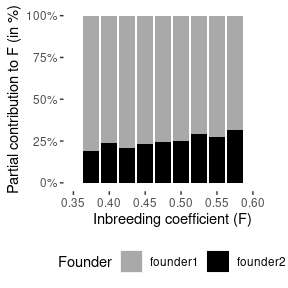Alternatively, partial inbreeding can also be estimated via genedrop simulation (using option genedrop). This will however result in less precise estimation of $$F_{i(j)}$$, and might only be convenient to use in terms of performance for very large and complex pedigrees. In these cases, a value of genedrop = 100 might give results that are well correlated with exact estimates ($$r > 0.9$$ for the pedigree examples provided).

### Ancestral inbreeding coefficient

The ancestral inbreeding coefficient ($$F_{a}$$, Ballou 1997) measures the probability of IBD of an individual for an allele that has been in homozygosity in at least one ancestor.

This parameter provides information not only about inbreeding, but can also be used to detect purging, since individuals with inbreeding $$F$$ and ancestral inbreeding $$F_{a}$$ are expected to be more fit than individuals with the same level of inbreeding but lower $$F_{a}$$, given that the ancestors of the former have survived and reproduced despite their higher inbreeding (see Boakes & Wang 2005 and López-Cortegano et al. 2018 for analyses using this parameter).

Ancestral inbreeding can be estimated for an individual $$i$$ with dam $$d$$ and sire $$s$$ as:

$F_{a_{i}} = \frac{1}{2}[F_{a_{d}} + (1-F_{a_{d}})F_{d} + F_{a_{s}} + (1-F_{a_{s}})F_{s}]$ Alternatively, a gene-dropping simulation approach can be used, following Baumung et al. (2015), providing unbiased estimates of $$F_{a}$$. This is because, above expression assumes that $$F$$ and $$F_{a}$$ are uncorrelated, which is not true.

Both approaches can be used with the function ip_Fa. Note that the computation of $$F_{a}$$ requires estimating $$F$$ in advance. Use argument Fcol to declare a column with $$F$$ values if it has been computed and saved in advance (this will save time), or leave it blank to compute it on the go.

# F was pre-computed above
darwin %>%
purgeR::ip_Fa(Fcol = "Fi") %>%
dplyr::filter(names == "William Erasmus Darwin")
#>   id dam sire                  names         Fi          Fa
#> 1 60  54   52 William Erasmus Darwin 0.06298828 0.001953125

# Compute F on the go (it won't be saved in the output)
# And enable genedropping
atlas %>%
purgeR::ip_Fa(genedrop = 1000, seed = 1234) %>%
dplyr::select(id, dam, sire, Fa) %>%
tail()
#>      id dam sire     Fa
#> 943 943 882  737 0.6475
#> 944 944 653  822 0.6000
#> 945 945 653  822 0.6000
#> 946 946 740  822 0.6050
#> 947 947 740  822 0.6075
#> 948 948 897  737 0.6340

$$F_{a}$$ can also be estimated based on population estimates of $$N_{e}$$ and generation numbers, using the expression from López-Cortegano et al. (2018):

$F_{a(t)} = 1 - (1-\frac{1}{2N})^{\frac{1}{2}t(t-1)}$ This can be achieved with the function exp_Fa (e.g. exp_Fa (Ne = 50, t = 50)).

### Purged inbreeding coefficient

The purged inbreeding coefficient ($$g$$) gives the probability of IBD for deleterious recessive alleles. The reduction of $$g$$ when compared to standard inbreeding depends on the magnitude of a purging coefficient ($$d$$) that measures the strength of the effective deleterious recessive component of the genome (García-Dorado 2012), so that $$d=0$$ implies $$F=g$$, and higher $$d$$ (up to 0.5) means lower $$g$$ in more inbred individuals. It can be calculated in pedigreed populations from the purged kinship coefficient ($$\gamma$$), in a similar way as standard inbreeding, following the methods described in García-Dorado (2012) and García-Dorado et al. (2016):

$\gamma_{i,i} = \frac{1}{2}(1+g_{i})(1-2dF_{i})$

$\gamma_{i,j} = \frac{1}{2}(\gamma_{i,j_{d}}+\gamma_{i,j_{s}})(1-dF_{j})$ Where $$j_{d}$$ and $$j_{s}$$ are $$j$$’s mother and father respectively, and $$i$$ is older than $$j$$.

The function ip_g computes the purged inbreeding coefficient, given a value of $$d$$. The choice of a proper value of $$d$$ can however be complex. A separate vignette titled “Inbreeding and Purging Estimates” describes methods to help computing the inbreeding load as well as the purging coefficient.

atlas %>%
ip_F() %>%
ip_g(d = 0.48, Fcol = "Fi") %>%
dplyr::select(id, dam, sire, Fi, tidyselect::starts_with("g")) %>%
tail()
#>      id dam sire        Fi      g0.48
#> 943 943 882  737 0.2350380 0.06066578
#> 944 944 653  822 0.2452226 0.08464775
#> 945 945 653  822 0.2452226 0.08464775
#> 946 946 740  822 0.2409467 0.07522799
#> 947 947 740  822 0.2409467 0.07522799
#> 948 948 897  737 0.2345642 0.06343757

$$g$$ can also be estimated based on population estimates of $$N_{e}$$ and generation numbers, given a value of $$d$$, using the expression from García-Dorado (2012):

$g_{t} = [(1-\frac{1}{2N})g_{t-1}+\frac{1}{2N}](1-2dF_{t-1})$

This can be achieved with the function exp_g (e.g. exp_g (Ne = 50, t = 50, d = 0.2)).

This is the last of functions related to inbreeding coefficients. Plotting together expected values of $$F$$, $$F_{a}$$ and $$g$$ (assuming $$N_{e} = 25$$ and an intermediate value $$d=0.25$$), the differences between the three coefficients become apparent.

data.frame(t = 0:50) %>%
dplyr::rowwise() %>%
dplyr::mutate(Fi = exp_F(Ne = 50, t),
Fa = exp_Fa(Ne = 50, t),
g = exp_g(Ne = 50, t, d = 0.25)) %>%
tidyr::pivot_longer(cols = c(Fi, Fa, g), names_to = "Type", values_to = "Inbreeding") %>%
ggplot(aes(x = t, y = Inbreeding, color = Type)) +
geom_line(size = 2) +
scale_x_continuous("Generations (t)") +
theme(legend.position = "bottom")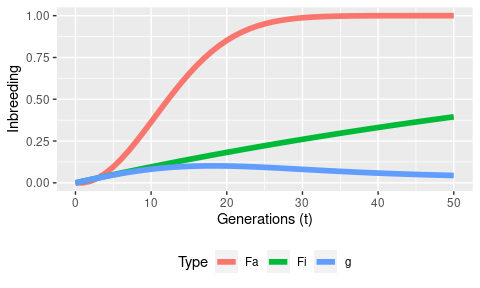### Opportunity of purging

Whereas previous purging methods focus on inbreeding measurements, opportunity of purging parameters calculate the potential reduction in individual inbreeding load, as a consequence of it having inbred ancestors (Gulisija and Crow 2007). The (total) opportunity of purging for an individual $$i$$ ($$O_{i}$$) can be computed as:

$O_{i} = \sum_{j}\sum_{k} (1/2)^{n-1} F_{j}$ Where $$j$$ is every inbred ancestor of $$i$$, $$k$$ is every path from $$i$$ to $$j$$, and $$n$$ is the number of individuals in the path (including $$i$$ and $$j$$).

The expressed opportunity of purging depends on the probability of a given allele to be transmitted from an inbred ancestor $$j$$ to $$i$$, and thus on $$F_{i(j)}$$. This is measured by the expressed opportunity of purging ($$O_{e}$$) as:

$O_{ei} = \sum_{j} 2F_{i(j)} F_{j}$ In complex pedigrees (involving more than one inbred ancestor per path), these measures need to be corrected to discount the probability of purging measured in a close ancestor from that already calculated in a more distant ancestor. The function ip_op(complex=TRUE) does not perform Gulisija and Crow (2007) corrections, but instead applies an heuristic approach, only accounting for close ancestors $$j$$, and ignoring contributions from far ancestors $$k$$ such that $$F_{j(k) > 0}$$.

The function ip_op can be used as:

arrui %>%
ip_op(Fcol = "Fi") %>%
dplyr::filter(target == 1) %>%
tidyr::pivot_longer(cols = c(Oe, Oe_raw)) %>%
ggplot() +
geom_point(aes(x = Fi, y = (value), fill = name), pch = 21, size = 3, alpha = 0.5) +
scale_y_continuous(expression(paste("Expressed opportunity of purging (", O[e], ")", sep=""))) +
scale_x_continuous("Inbreeding coefficient (F)") +
scale_fill_discrete("")
#> Computing partial kinship matrix. This may take a while.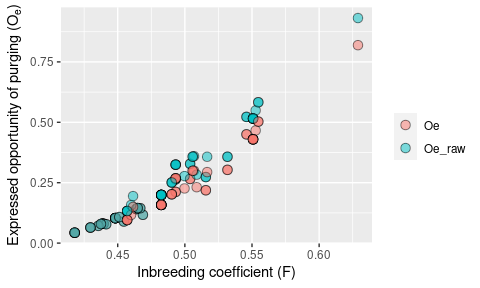The plot shows the increase of the expressed opportunity of purging with the inbreeding coefficient for individuals in the reference population (last two cohorts). For individuals with the lowest inbreeding values, the corrected ($$O_{e}$$) and uncorrected ($$O_{e \_raw}$$) have the same value, but as time progresses $$O_{e \_raw}$$ becomes larger than $$O_{e}$$. Both values are useful when determining potential reduction in the individual inbreeding load (see more exhaustive examples in López-Cortegano 2021, and the ‘Inbreeding and Purging Estimates’ vignette).

## Population parameters

The package purgeR is mainly focused on estimating inbreeding and purging parameters, but accessory functions are included to compute other population parameters that might be useful when interpreting inbreeding and purging results. All functions for computing population parameters are prefixed with pop_.

### Effective population size

The effective population size ($$N_{e}$$) can be computed from the individual increase in inbreeding ($$\Delta F$$) as defined by Gutiérrez et al. (2008, 2009):

$N_{e} = \frac{1}{2\Delta F}$ Where the individual $$\Delta F$$ can be computed as:

$\Delta F_{i} = 1 - \sqrt[t_{i}-1]{1-F_{i}}$ Being $$F_{i}$$ individual’s i coefficient of inbreeding, and $$t_{i}$$ the generation number it belongs to.

The previous expression can be averaged to obtain $$\Delta F$$ and used to estimate $$N_{e}$$. Thus, all that is needed to compute $$N_{e}$$ in a pedigree is the individual values of inbreeding and generation time. The function pop_Ne will read a pedigree file and calculate $$N_{e}$$ using accessory columns containing inbreeding and time information (named ‘F’ and ‘t’ here). Note that the generation number is estimated here with pop_t as the number of equivalents to complete generations (see below).

atlas %>%
purgeR::ip_F() %>%
purgeR::pop_t() %>%
purgeR::pop_Ne(Fcol = "Fi", tcol = "t")
#> $Ne #>  8.184803 #> #>$se_Ne
#>  0.2498695

However, we must warn caution when estimating $$N_{e}$$ this way. Note that the previous estimate includes all individuals in the pedigree, but only the most recent individuals should be used, as they already account for the inbreeding in their ancestors. In the following data set, the column target indicates the individuals that belonged to the reference population used to estimate $$N_{e}$$ (see details in López-Cortegano et al. 2021). Thus, $$N_{e}$$ should be estimated in this case as:

atlas %>%
purgeR::ip_F() %>%
purgeR::pop_t() %>%
dplyr::filter(target == 1) %>%
purgeR::pop_Ne(Fcol = "Fi", tcol = "t")
#> $Ne #>  14.01041 #> #>$se_Ne
#>  0.1707653

It is worth mentioning that this method to estimate $$N_{e}$$ is of course equivalent to that using the classical formula $$F=1-(1-\frac{1}{2N_{e}})^t$$.

### Number of equivalents to complete generations

Generation times are easily computed for populations with discrete generations, but overlapping generations are the rule in most real world populations, and methods are required to estimate generation times in such circumstances. The function pop_t computes the number of equivalents to complete generations ($$t_{eq}$$) following Boichard et al (1997). This is calculated for an individual i as:

$t_{eq} = \sum^{J}_{j=1} (\frac{1}{2})^{n}$ Where the sum is over all known ancestors, and $$n$$ is the number of discrete generations that separate individual i from its ancestor j.

Of course, in populations with discrete generations, $$t_{eq} = t$$, and in those with overlapping generations the estimates of $$t_{eq}$$ strongly correlates with time. Consider as an example the plot below showing the increase of $$t_{eq}$$ with the year of birth ($$yob$$) of Gazella cuvieri:

atlas %>%
purgeR::pop_t() %>%
dplyr::mutate(t = plyr::round_any(t, 0.5)) %>%
ggplot() +
geom_boxplot(aes(x = yob, y = t, group = yob)) +
scale_y_continuous(expression(t[eq]))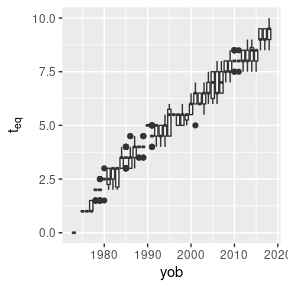Not only is the correlation strong, but the total number of generations estimated (~10) matches the expectation given the total number of years of management (45) and the mean age for breeding females (4.31 years, Moreno and Espeso 2008).

### Number of founders and ancestors

Functions are also included to compute the total and effective number of founders and ancestors, as well as the number of founder genomes equivalents ($$N_{g}$$). These parameters can provide information on early population bottlenecks due to unbalanced founder or ancestor contributions, as well as drift. Their estimation is based on probability of gene origin computations, following Boichard et al (1997), but Caballero and Toro (2000) and Tahmoorespur and Sheikhloo (2011) are also recommended lectures in this regard. All these parameters are referred to a reference population (RP) of interest that must be defined, e.g. it could be the latest cohort, or even the entire population.

The total number of founders ($$N_{f}$$) is calculated simply as the count of founders of the RP, while the effective number of founders ($$N_{fe}$$) is the number of equally contributing founders that account for the observed genetic diversity in the RP. Founders are defined as individuals with not known parents (i.e. dam = 0 and sire = 0).

The total number of ancestors ($$N_{a}$$) is the count of all ancestors that contribute descendants to the RP, founders or not, while the effective number of ancestors ($$N_{ae}$$) is calculated as the minimum number of ancestors, founders or not, required to account for the genetic diversity observed in the RP.

The number of founder genome equivalents ($$N_{g}$$) is defined in a similar way as ($$N_{fe}$$), but its estimated via Monte Carlo simulation of allele segregation, effectively accounting not only for reductions in genetic diversity as consequence of bottlenecks in founders or ancestors contributions to the descent, but also to random sources of diversity loss, such as drift (Boichard et al 1997, Caballero and Toro 2000).

Thus, $$N_{ae}$$ is always smaller than $$N_{f}$$, and their ratio can inform on the diversity loss due to bottlenecks between the base population and the RP (Tahmoorespur and Sheikhloo 2011). On the other hand, $$N_g$$ is always the smallest parameter among these, since it accounts not only for diversity loss due to unbalanced founder or ancestor contributions, but also to genetic drift.

The function pop_Nancestors computes all these parameters, and returns them in a dataframe:

list("A. lervia" = arrui,
"G. cuvieri" = atlas,
"G. dorcas" = dorcas,
"N. dama" = dama) %>%
purrr::map_dfr(~ pop_Nancestors(., reference = "target", seed = 1234), .id = "Species")
#>      Species  Nr Nf       Nfe  Na       Nae       Ng     se_Ng
#> 1  A. lervia  80  2  1.769424  63  1.769424 1.034102 0.2468827
#> 2 G. cuvieri 176  4  3.583086 249  3.583086 2.002087 0.4601456
#> 3  G. dorcas 283 20 13.386468 400 12.990604 5.817682 1.0154111
#> 4    N. dama 251  4  2.614750 349  2.614750 1.868039 0.4506204

Convenience functions are also available, named after the parameters they estimate. For example, the function pop_Ng will just estimate the number of founder genome equivalents, and return that value as a numeric value. Similarly, pop_Nae will only estimate the effective number of ancestors, and so on. See more examples in ?pop_Nancestors.

atlas %>% purgeR::pop_Ng(reference = "target", seed = 1234)
#> $Ng #>  2.002087 #> #>$se_Ng
#>  0.4601456
atlas %>% purgeR::pop_Nae(reference = "target")
#>  3.583086

### Hardy-Weinberg deviation

In some cases it might be of interest to measure the degree of non-random mating in the population. This is given by deviation from Hardy-Weinberg equilibrium ($$\alpha$$, Caballero and Toro 2000), that can be calculated as:

$\alpha = \frac{F-f}{1.0-f}$ Where $$F$$ is the mean inbreeding coefficient of the population, and $$f$$ the mean coancestry coefficient.

The function pop_hwd allows to estimate the previous coefficient, for the entire population, or preferably for a RP:

atlas %>% purgeR::pop_hwd(reference = "target")
#>  -0.02753781

Note that in the example above $$\alpha$$ is negative, as usually attributed to populations undergoing management. A value of zero would indicate random mating, and a positive one assortative mating among relatives.

## Fitness functions

Purging analyses may benefit from an interpretation in terms of fitness change. Fitness measurements are expected to be provided by the users, and could be for example ‘early survival’, or any other trait known to be related to fitness in the studied species. A small set of functions is given however to help users to infer fitness measurements from the pedigree structure itself.

We warn, however, to make use of these with caution, as they might not always reflect true fitness. First, because measures of fitness based on contributions to the offspring (usually named as ‘breeding success’ or ‘productivity’) are limited to individuals present in the pedigree, and that information could be incomplete; Second, if the population is under active management, offspring contributions may not represent actual biological fitness; Third, ‘reproductive values’ give expectations based on additive genetic relatedness and do not account for selective effects. Thus, they may be unappropriated for downstream analysis considering purging effects; Finally, younger individuals in the pedigree might have lower fitness than older ones, because they haven’t had time to generate offspring!

Fitness functions are prefixed with w_. A first measure of fitness given by the pedigree is individual breeding success, measured as the number of offspring present in the pedigree. The function w_offspring can be used for this:

# Maximum overall breeding success
arrui %>%
purgeR::w_offspring(name_to = "P") %>%
.$P %>% max() #>  69 # Maximum female breeding success arrui %>% purgeR::w_offspring(name_to = "P", sire_offspring = FALSE) %>% .$P %>%
max()
#>  21

Similarly, the number of grandoffspring can also be used as a proxy for fitness, with the function w_grandoffspring:

# Maximum overall grandoffspring productivity
arrui %>%
purgeR::w_grandoffspring(name_to = "GP") %>%
.\$GP %>%
max()
#>  198

Finally, fitness can also be estimated as ‘reproductive values’, following the method developed by Hunter et al. (2019). Under this model, fitness is based on how well a gene originated in a set of reference individuals is represented in their descendants. We do not go into the details of the algorithm used in this model, but it allows to correct genetic contributions by changes in population size and migration (which is used by default). This method can be used with the function w_reproductive_value:

dama %>%
purgeR::pop_t() %>%
dplyr::mutate(t = plyr::round_any(t, 1), t = as.integer(t)) %>%
purgeR::w_reproductive_value(reference = "t", name_to = "R", generation_wise = TRUE) %>%
dplyr::filter(t != max(t)) %>%
ggplot() +
geom_boxplot(aes(x=factor(t), y=R)) +
scale_x_discrete("t")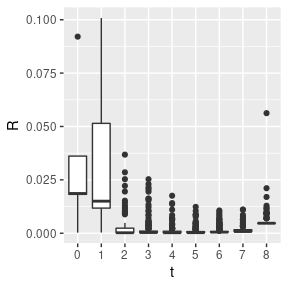## Other functions

### Maternal effects

Sometimes it can be useful to assign a given individual $$i$$ its maternal inbreeding coefficient, or any other value, for example to evaluate maternal effects. The function ped_maternal will read one of the columns present in the pedigree data frame, and assign to every individual the value observed in their mothers (or fathers if use_dam = FALSE option is used). For individuals with unknown parents, NA values will be returned by default, but this can be overridden with the option set_na.

arrui %>%
purgeR::ped_maternal(value_from = "Fi", name_to = "Fdam") %>%
dplyr::filter(id %in% c(317, 380)) %>%
dplyr::select(id, dam, sire, Fi, Fdam)
#>    id dam sire        Fi      Fdam
#> 1 317 293  275 0.4384766 0.5468750
#> 2 380 317  303 0.4902344 0.4384766